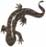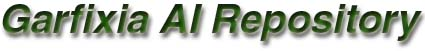There are neural networks in all sorts and sizes. I attempted to create a framework to facilitate the creation of a wide variety of concrete neural networks. The framework that is presented here is capable of creating most of the neural networks as described by McClelland and Rumelhart in their "Explorations in parallel distributed processing". Even though the framework is generic, it is still capable of creating only a small subset of the existing neural networks. For now I will leave it at that, with the intention to expand its capability in the future.
The framework attempts to find a balance between proper object oriented, extendible design, which is so often lacking in neural network implementations, and calculation speed, which is essential in practical applications. So I believe the framework is both well designed and fast. However, a hardwired implementation of any neural network is always faster than any generic design can offer. But if maintainability and clarity of design are requirements of the task, this framework is a serious option.

What was implemented:
• The main structure is the model (CNNModel). The model contains pools (CNNPool) and connections between pools (CNNPoolConnection). Each pool contains units (CNNUnit). Each pool connection contains all connections between the units of one pool and another. This setup allows for an explicit modeling of the main structure of the neural network. Its very easy to create a populated pool and to create a relationship between two pools. Specifying the characteristics of the relationship (like excitation and inhibition parameters) can be made explicitly and easily.
• The following parameters are implemented: external input, rest, decay, minimum, maximum, threshold, bias, alpha, gamma, estr, istr.
• These update methods are implemented: Linear, Linear Threshold, Grossberg, Stochastic, and Continuous Sigmoid.
• These training methods are implemented: Hebbian, Generalized Delta Rule.
What was not implemented:

It's obvious that it is a major undertaking, if at all possible, to design a framework that is capable of creating all possible neural networks. Here are some things I didn't implement from the McClelland and Rumelhart workbook:

• Harmony Theory. Because it looked too farfetched to me. Maybe if the problems it can solve cannot be solved in another way, it can be worthwhile implementing it. Otherwise it only seems to introduce a lot of funny parameters.
• Updating weights per epoch. Weights can only be updated per pattern, not per epoch. This made the code easier to read, made the units less heavy, and it made the application code easier. All this while the performance should be about the same, perhaps even better.
• In the same vain, I chose not to model the number of patterns to be learned explicitly, since I expect that the number of patterns is usually unlimited in real life. Thus, there is no TSS (total sum of squares), but only a PSS (pattern sum of squares).
Remarks
• At first I tried to model every single connection between two units as a separate object. This seemed like a good design, especially for sparsely distributed relations. However, in practice, most networks use fully connected pools. The implementation caused both a major impact on memory requirements and on the time it took to build the network (creating millions of objects...). Therefore I now place all connections between two pools in a single two dimensional weight array, as is the most common way to do it. This means that whenever two pools are connected, they are fully connected. When two units are not meant to be connected, there weight is set to zero.
• I found out (the hard way, by studying c source files for days) that M&R calculate the goodness of their constraint satisfaction network not by adding up the goodnesses of individual units, but, since the constraints (represented by connections between units) are non-directional, they use only the weight A->B, but not the weight B->A in their calculation. I decided not to follow them here, since they wrote in their book that the collective goodness should equal the summed goodness, and by using all weights the architecture becomes more general (it also allows for directional constraints) and simpeler.
• McClelland and Rumelhart chose to present input patterns (which equal the target patterns) of autoassociators via external input fields. They could also have chosen for the activation or target fields. I have chosen to present input patterns via activation fields, since it is the standard way to do it in backpropagation learning, and that seemed a good standard. Furthermore, it is somewhat easier to implement.
Sample use

This code implements a simple XOR model, a three layer model that is trained by backpropagation.

```#include <conio.h>
#include "aiNNModel.h"
#include "genericArray.h"

/// The XOR model, which is a 3 layer network, trained by backpropagation
/// to act as an xor logical gate.
///
/// from "Explorations in Parallel Distributed Processing" (chapter 5)
/// by McClelland & Rumelhart

#define EPOCHCOUNT 300
#define PATTERNCOUNT 4
// error criterion (4 times 0.1 squared)
#define ECRIT 0.04f

void Xor(void)
{
CNNModel XorModel;
CArray<float> SourcePatterns[PATTERNCOUNT];
CArray<float> TargetPatterns[PATTERNCOUNT];
float TotalSumOfSquares = 1.0f;
int EpochIndex;

// create pools

Inputs->SetPoolType(POOLTYPE_INPUT);
Hiddens->SetPoolType(POOLTYPE_HIDDEN);
Outputs->SetPoolType(POOLTYPE_OUTPUT);

// create connections between pools, interconnect all units

// pool parameters
Hiddens->SetUpdateMethod(UPDATEMETHOD_CONTINUOUS_SIGMOID);
Outputs->SetUpdateMethod(UPDATEMETHOD_CONTINUOUS_SIGMOID);

// randomize weights and biases (to break symmetry)
I2HConnection->RandomizeWeights();
H2OConnection->RandomizeWeights();
Hiddens->RandomizeBiases();
Outputs->RandomizeBiases();

// train the model
XorModel.SetTrainingMethod(TRAININGMETHOD_GENERALIZED_DELTA);
XorModel.SetLearningRate(0.5f);
for (EpochIndex=0; EpochIndex<EPOCHCOUNT; EpochIndex++)
{
TotalSumOfSquares = 0.0f;
for (int PatternIndex=0; PatternIndex<PATTERNCOUNT; PatternIndex++)
{
Inputs->SetOutputPattern(SourcePatterns[PatternIndex]);
Outputs->SetTargetPattern(TargetPatterns[PatternIndex]);
XorModel.TrainPattern();
TotalSumOfSquares += Outputs->GetPatternSumOfSquares();
}
// total error below error criterion?
if (TotalSumOfSquares < ECRIT) break;
}
printf("Epochs used (needed): %d\n\n", EpochIndex+1);

// check the results
XorModel.SetUpdateOrder(UPDATEORDER_HIDDEN_OUTPUT);
for (int PatternIndex=0; PatternIndex<PATTERNCOUNT; PatternIndex++)
{
// set input pattern
Inputs->SetOutputPattern(SourcePatterns[PatternIndex]);

// update all units
XorModel.Update();

// print results
printf("%d xor %d = %f\n",
(int)SourcePatterns[PatternIndex].Get(0),
(int)SourcePatterns[PatternIndex].Get(1),
(Outputs->GetUnit(0)->GetOutput())
);
}
}                ```